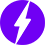As an Amazon Associate I earn from qualifying purchases.

# Construction. Working, Connections & Applications

We all must have witnessed and used a cylindrical device called the rheostat while performing experiments in the Physics lab. But we never really delved into its technicalities.

A rheostat is a type of variable resistor, whose resistance can be changed for varying the amount of electric current flowing through an electrical circuit. Commonly available resistors have a fixed value and are used for restricting smaller values of electric current. Rheostat is used for varying higher values of electric current.

## Brief History

In the nineteenth century, Sir Charles Wheatstone invented the rheostat using a long tube with coiled wires around it and an adjustable slider. The word rheostat is made of two words (‘rheo’ meaning flow of current in Greek and ‘stat’ meaning stationary instrument). When placed in an electric circuit, the flow of electricity changed through two terminals: one terminal near the slider/adjustable contact and the other connected near the bottom.## Construction

The modern day rheostat is not much different from its earlier version. A long cylindrical structure having a ceramic core has Nichrome wires tightly wound around it.The ceramic core acts as an insulating material to the generated heat.

Similar to a potentiometer, the rheostat has three terminals out of which only two are used. A slider is present at the top, which can freely move and is in contact with the wounded wires.

## Working Principle

A rheostat is based on Ohm’s law, which is given by:

R = V/I

where R= resistance
V= voltage
I= current

From the above law, we can see that resistance is inversely proportional to current. This means that an increase in resistance decreases the current and vice-versa.

Also, according to the following formula:

R = ρL/A

where R = resistance
ρ = resistivity
L = length
A = area of cross-section

resistance is directly proportional to length. Therefore, resistance increases as the length of the wire (i.e. number of turns) increases.

## Connections

As stated earlier, out of three terminals of a rheostat, only two are used.

The above diagram shows how connections are made in a rheostat when placed in an electrical circuit. One end of the wire from where current enters the device is connected to the bottom-left terminal (terminal A). By moving the wiper/slider, the resistance can be increased or decreased. This varied current then flows out through the top-right terminal (terminal B) further into the electrical circuit.

The wiper/slider being close to terminal A indicates low resistance whereas it increases when close to terminal B.

If we use terminals B and C, a minimum resistance is achieved when we move the wiper/slider close to the terminal B because the length of the resistive path is now decreased. This leads to a flow of large amounts of electric current. When the wiper/slider is moved to terminal A, maximum resistance is achieved as the length of the resistive path increases. Therefore, large flow of electric current gets restricted.

A rheostat is internationally denoted by the following symbol:

## Applications

Rheostat is generally used in applications where high voltage or current is required such as:

• Changing the light intensity of a light bulb. An increase in the resistance of the rheostat decreases the flow of electric current, leading to dimming of lights and vice-versa.
• Generators
• Motor speed
• Heater and oven temperature control
• Volume control

## Types of rheostat

Linear: It is cylindrical in shape in which the wiper or slider moves in a linear manner. It has a linear resistive path. These are mostly used in laboratories for teaching and experiments.Rotary: It has a rotary resistive path. In this, the wiper or slider is mounted on a shaft and moved in a rotary manner, rotating over 3⁄4 of a circle. These are mostly used in power applications.Preset: These are small in size and are nothing but a small rheostat. Trimmers or preset rheostats are used in a printed circuit board for calibration purposes.## Rheostat versus potentiometer

Although they both serve the purpose of varying the amount of resistance, they have certain dissimilarities.

 Rheostat Potentiometer 2-terminal device; two terminals used for the operation 3-terminal device; three terminals used for the operation Cannot be utilised like a potentiometer Can be utilised like a rheostat Changes the current Changes the voltage